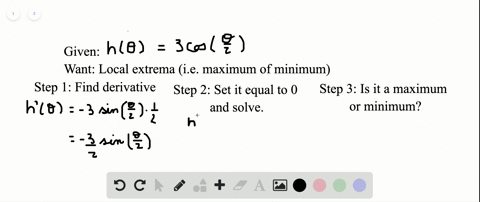Enroll in one of our FREE online STEM bootcamps. Join today and start acing your classes!View Bootcamps### Show that the functions have local extreme values…

04:16Georgia Southern University
Problem 62

# The graph of $f^{\prime}$ is given. Assume that $f$ is continuous and determine the $x$ -values corresponding to local minima and local maxima.

## Discussion

You must be signed in to discuss.

## Video Transcript

guys. I'm looking at the graph of the derivative and I know as we'Ll have a local Maxima when eth prime goes from being positive too negative and then a minima when f prime Because from negative post. Okay, so the derivative is almost always positive except between X equals zero and X equals two. And we see that the only time the derivative changes sign is at zero and two. So it looks like because at X equals zero s prime changes from being positive to negative. So we have a a little cool max in the next sequels to F prime changes from negative. Positive. So you have a Oh, cool. No, Isn't it too? And now we do not know what the values of these local Knox and men are. They could be shifted by a constant between different functions that have the same drift. But we know that we have a local max zero and local minute, too. Because of the derivative changing sign